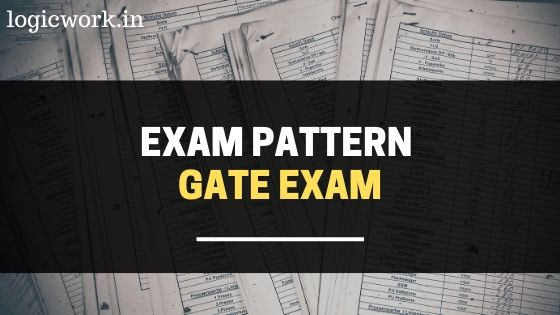# GATE 2021 Exam Pattern

## GATE Exam Pattern & Marking Scheme

• The Gate Exam will have 2 types of Questions- Multiple Choice Questions (MCQ) Numerical Answer Type (NATQuestions.
• The duration of all the GATE Exam will be of 3 hours.
• The entire paper will be divided into three sections viz.
• Section A – General Aptitude,
• Section B – Engineering Mathematics and
• Section C –  Subject paper.

## GATE Exam Pattern

### GATE Exam Pattern – (AE, AG, BT, CE, CH, CS, EC, EE, IN, ME, MN, MT, PE, PI, TF, and XE)

• Engineering mathematics questions are mandatory and carry 15 % of the whole weightage (15 Marks).
• The questions based on the topic of the exam have 70% of the whole weightage (70 Marks).
• The remaining a part of the GATE Question Paper includes General aptitude queries (15% of the whole weightage – fifteen Marks)

### GATE Exam Pattern – (AR, CY, EY, GG, MA, PH, and XL )

• The general aptitude section can carry 15 of the full marks (15 Marks).
• The remaining 85% of the total marks are based on the subject of the paper (85 Marks).
• There is no question from Engineering mathematics in these papers.
• NAT includes questions with a value or a real number as their answer.
• NAT questions are mentioned with, up to which decimal places, the candidates have to be compelled to make a solution.
• The answer must be entered using the mouse.
• There won’t be any choices for these questions.
• Using the keyboard should be avoided as it can lock your answer panel.
• There will be no negative marking for NAT questions.

### GATE Marking Scheme

For each of the 25 subjects, the paper consists of 65 questions of 100 marks. Every paper is split into subsections and on the basis of it, marking theme is outlined.

• From a total of 100 marks, there will be 10 questions of 15 marks covering the entire General Aptitude section.
• General Aptitude- 5 Questions of 2 Marks each and 5 Questions of 1 Marks each.
• Engineering Mathematics will cover approximately 15% weightage out of 100 marks for the paper bearing codes AE, AG, BT, CE, CH, CS, EC, EE, IN, ME, MN, MT, PE, PI, TF, and XE.
• Engineering Mathematics- 5 Questions of 2 Marks each and 5 Questions of 1 Marks each.
• Remaining 70% weightage of total marks is acquired by the subject of the paper.
• Subject Knowledge- 25 Questions of 2 Marks each and 20 Questions of 1 Marks each.
• For the paper codes – AR, CY, EY, GG, MA, PH and XL, the General Aptitude will cover 15% out of 100 marks, and the other 85% of the total question paper will be covered by the subject of the paper.

### Negative Marking in GATE Exam Pattern

• There is no provision of negative marking in NAT questions.
• MCQs carrying 1 marks each, 0.33 marks would be deducted as a penalty of marking the wrong answer.
• For questions carrying 2 marks, 0.66 marks would be deducted for marking an incorrect answer.